RRB NTPC Mock Test - 16 (English)

# RRB NTPC Mock Test - 16 (English)

Test Description

## 100 Questions MCQ Test RRB NTPC Mock Tests Series | RRB NTPC Mock Test - 16 (English)

RRB NTPC Mock Test - 16 (English) for Railways 2023 is part of RRB NTPC Mock Tests Series preparation. The RRB NTPC Mock Test - 16 (English) questions and answers have been prepared according to the Railways exam syllabus.The RRB NTPC Mock Test - 16 (English) MCQs are made for Railways 2023 Exam. Find important definitions, questions, notes, meanings, examples, exercises, MCQs and online tests for RRB NTPC Mock Test - 16 (English) below.
Solutions of RRB NTPC Mock Test - 16 (English) questions in English are available as part of our RRB NTPC Mock Tests Series for Railways & RRB NTPC Mock Test - 16 (English) solutions in Hindi for RRB NTPC Mock Tests Series course. Download more important topics, notes, lectures and mock test series for Railways Exam by signing up for free. Attempt RRB NTPC Mock Test - 16 (English) | 100 questions in 90 minutes | Mock test for Railways preparation | Free important questions MCQ to study RRB NTPC Mock Tests Series for Railways Exam | Download free PDF with solutions
 1 Crore+ students have signed up on EduRev. Have you?
RRB NTPC Mock Test - 16 (English) - Question 1

### Select the related word from the given alternatives. Ally : enemy :: ?

Detailed Solution for RRB NTPC Mock Test - 16 (English) - Question 1

Ally means to merge or friend, opposite of enemy, likewise ancient is opposite of Modern.

Hence, Ancient : Modern is the correct answer.

RRB NTPC Mock Test - 16 (English) - Question 2

### The processor speed of a computer is measured in________.

Detailed Solution for RRB NTPC Mock Test - 16 (English) - Question 2
• The processor speed of a computer is measured in MIPS because the processor works to give instructions.
• MIPS stands for Million Instructions Per Second.
• BPS stands for Bits Per Second. It is a standard way to measure data transfer rates.
• Baud is used to describe the maximum oscillation rate of an electronic signal.
• Hertz is the standard unit of measurement used for measuring frequency.
RRB NTPC Mock Test - 16 (English) - Question 3

### Chucker is related to ________.

Detailed Solution for RRB NTPC Mock Test - 16 (English) - Question 3
• Chucker is related to Polo.
• Chucker is a term used in Polo to define a time segment like halves or quarters in other games like football.
• The normal duration of chukka/chucker is 7-minutes after 7 minutes of play continues for an additional 30 seconds or until a stoppage in play.
• There are six or more chucker in one polo match.
RRB NTPC Mock Test - 16 (English) - Question 4

The speed of a boat in still water is 50 km/hr. It covers a distance of 500 km upstream in 20 hours. What is the speed (in km/hr) of the stream?

Detailed Solution for RRB NTPC Mock Test - 16 (English) - Question 4

Let the speed of the stream = x km/hr

Given,

Speed of the boat in still water = 50 km/hr

∴ Speed of the boat towards upstream = (50 - x) km/hr

Distance covered = 500 km

Time taken = 20 hr

∵ Distance covered = Speed × Time taken

⇒ 500 = (50 - x) × 20

⇒ 25 = (50 - x)

⇒ x = 25 km/hr

RRB NTPC Mock Test - 16 (English) - Question 5

Who among the following was the author of the book “Kamayani”?

Detailed Solution for RRB NTPC Mock Test - 16 (English) - Question 5
• Jaishankar Prasad was the author of the book “Kamayani”.
• Kamayani is a Hindi epic poem.
• It is considered one of the great literary works written in modern times in Hindi Literature.
• It also signifies the epitome of Chhayavai school of Hindi poetry gained popularity in the late 19th and early 20th centuries.
RRB NTPC Mock Test - 16 (English) - Question 6

In the following question below are given some statements followed by some conclusions. Taking the given statements to be true even if they seem to be at variance from commonly known facts, read all the conclusions and then decide which of the given conclusion logically follows the given statements.

Statements: I. All blue are black. II. Some blue are yellow.

Conclusions: I. All blue are yellow. II. Some black are yellow.

Detailed Solution for RRB NTPC Mock Test - 16 (English) - Question 6

Consider the following least possible Venn diagram,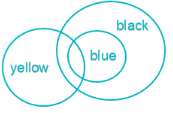Conclusions:

I. All blue are yellow → False (It is possible but not definite).

II. Some black are yellow → True (It is definitely true as the part of blue which are yellow is also black as all blue are black).

Hence, only conclusion II follows.

RRB NTPC Mock Test - 16 (English) - Question 7

Jharkhand was formed as the which state of the country?

Detailed Solution for RRB NTPC Mock Test - 16 (English) - Question 7
• On 15 November 2000 Jharkhand became the 28th state of India, separated from Bihar.
• On 9 November 2000 Uttarakhand became the 27th state of India and was separated from Uttar Pradesh.
• On 1 November 2000 Chhattisgarh became the 26th state of India and was separated from Madhya Pradesh
• On 2 June 2014 Telangana was separated from the Andhra Pradesh to form the newly formed 29th state.
RRB NTPC Mock Test - 16 (English) - Question 8

The average marks obtained by 120 candidates in a certain examination is 35. If the average mark of passed candidates is 39 and that of the failed candidates is 15, what is the number of candidates who passed the examination?

Detailed Solution for RRB NTPC Mock Test - 16 (English) - Question 8

Total number of candidates = 120

Let the number of candidates who passed the exam be = x

No. of failed candidates = (120 - x)

Then total marks = 120 × 35 = 39x + (120 - x) × 15

⇒ 4200 = 39x + 1800 - 15x

⇒ 4200 - 1800 = 24x

∴ x =  2400/24  = 100

RRB NTPC Mock Test - 16 (English) - Question 9

A series is given with one term missing. Select the correct alternative from the given ones that will complete the series.
93, 102, 110, _______, 123, 128, 132

Detailed Solution for RRB NTPC Mock Test - 16 (English) - Question 9

The relation among the given numbers is:

93 + 9 = 102

102 + 8 = 110

110 + 7 = 117

117 + 6 = 123

123 + 5 = 128

128 + 4 = 132

Hence, “117” is the missing term.

RRB NTPC Mock Test - 16 (English) - Question 10

Which of the following country marks its entry into space after the launch of its first satellite named as ‘Raavana-1’?

Detailed Solution for RRB NTPC Mock Test - 16 (English) - Question 10
• Sri Lanka’s first satellite ‘Raavana-1’ was launched into space from NASA’s Flight Facility on Virginia’s east shore.
• The satellite was designed and built at the Kyushu Institute of Technology in Japan by two Sri Lankan research engineers.
• Its camera mission is to capture images of Sri Lanka and its neighboring countries.
RRB NTPC Mock Test - 16 (English) - Question 11

Select the jumbled word which is different from the other three alternatives.

Detailed Solution for RRB NTPC Mock Test - 16 (English) - Question 11

1) MELAC → CAMEL

2) RITEG → TIGER

3) ROSHE → HORSE

4) UFBAFOL → BUFFALO

Except 'Tiger' all are herbivores whereas 'Tiger' is a carnivore.

Hence, 'Tiger' is the odd one.

RRB NTPC Mock Test - 16 (English) - Question 12

The ratio of the sixth and the seventh terms of the sequence 1, 3, 6, 10, ___

Detailed Solution for RRB NTPC Mock Test - 16 (English) - Question 12

Difference of consecutive terms: 2,3,4,5,6,7,8……

We can see that the difference of consecutive terms in the sequence are in AP with first term 2 and common difference = 1.

∴ nth term of this sequence (1,3,6,10,……) is given as, a_n = a_(n - 1) + (2 + (n - 2)d), n ≥ 2.

Now, a5 = a4 + (2 + (5 - 2)1) = 10 + (2 + 3) = 10 + 5 = 15

a6 = a5 + (2 + (6−2)1) = 15 + (2+4) = 15  + 6 = 21
a7 = a+ (2 + (7−2)1) = 21+(2+5) = 21 + 7 = 28

Now, a6/a7 = 21/28 = 3/4

⇒ a6:a7=3:4

RRB NTPC Mock Test - 16 (English) - Question 13

Gerontology is known as the study of __________.

Detailed Solution for RRB NTPC Mock Test - 16 (English) - Question 13
• Gerontology is known as the study of old age.
• Gromatici is known as the science of surveying.
• Gynaecology is known as the study of women’s physiology.
• Gyrostatics is known as the study of rotating bodies.
RRB NTPC Mock Test - 16 (English) - Question 14

Direction: A statement is given followed by two inferences I and II. You have to consider the statement to be true even if it seems to be at variance from commonly known facts. You have to decide which of the given inferences if any follow from the given statement.

Statement: Rajiv Sharma, dissatisfied with his present salary and perks, joins another company.

Inference:
I. The new company gives all its employees a good salary.
II. The present company runs in loss.

Detailed Solution for RRB NTPC Mock Test - 16 (English) - Question 14

Option 4 is the correct answer. As we see in the statement, Rajiv Sharma is dissatisfied with his salary and perks, So he joins another company. The inference I is about the new company giving good salary to all its employees, which is not mentioned in the statement. Hence, I does not follow here. The inference II talks about present company's condition about which no information is given in the statement. So, both inferences do not follow.

RRB NTPC Mock Test - 16 (English) - Question 15

Which among the following is a coordination bond?

Detailed Solution for RRB NTPC Mock Test - 16 (English) - Question 15
• Carbon monoxide is formed by a coordinate bond.
• A covalent bond is formed by two atoms sharing a pair of electrons.
• A coordinate bond is a type of covalent bond where both of the electrons that form the bond originate from the same atom.
• Carbon monoxide has two ordinary covalent bonds between the carbon and oxygen and also a coordinate bond using a lone-pair on the oxygen atom.
RRB NTPC Mock Test - 16 (English) - Question 16

Select the option that is related to the third term in the same way as the second term is related to the first term.
Work : Joule ∷ Power : ______

Detailed Solution for RRB NTPC Mock Test - 16 (English) - Question 16

SI unit of Work is Joule.

Similarly, SI unit of Power is Watt.

Hence Power is related to Watt.

RRB NTPC Mock Test - 16 (English) - Question 17

Britisher Charles II got Bombay as dowry For marrying which of the following princess?

Detailed Solution for RRB NTPC Mock Test - 16 (English) - Question 17
• The king of England Charles II got Bombay as dowry for marrying Catherine in 1661 AD.
• According to the marriage treaty of Charles II of England and Catherine of Braganza, daughter of King John IV of Portugal on 21 May 1662, placed Bombay in possession of the British Empire.
• Currently, Bombay is the capital city of Maharashtra.
• It is also known as the financial capital of India.
• According to the 2011 census, It was the most populous city of India.
RRB NTPC Mock Test - 16 (English) - Question 18

Which among the following is a fibre protein?

Detailed Solution for RRB NTPC Mock Test - 16 (English) - Question 18
• Keratin is a fibre protein. This protein is found in hair, nail, horn, wool etc.
• It also protects epithelial cells from damage or stress.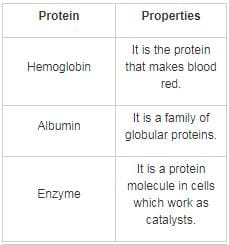RRB NTPC Mock Test - 16 (English) - Question 19

What is the value of [(a-2b3) ÷ (a1b-1)] × [(a2b-4) ÷ (a-1b2)]?

Detailed Solution for RRB NTPC Mock Test - 16 (English) - Question 19

[(a-2b3) ÷ (a1b-1)] × [(a2b-4) ÷ (a-1b2)]

Using BODMAS rule am ÷ an = am - n

[(a-2b3) ÷ (a1b-1)] × [(a2b-4) ÷ (a-1b2)]

= a-2 - 1b3-(-1) × a2-(-1)b-4 - 2

a-3b4 × a3b-6

am × an = am + n

= a-3 + 3b4 - 6

= b-2

= 1/b2

RRB NTPC Mock Test - 16 (English) - Question 20

In a right angled triangle if sinB = (5/13) then find the value of tanB + cosecB.​

Detailed Solution for RRB NTPC Mock Test - 16 (English) - Question 20

Given sinB = (5/13) = Perpendicular/hypotenuse

⇒ We know that (Hypotenuse)2 = (Perpendicular)2 + (Base)2

⇒ (13)2 = (5)2 + (Base)2

⇒ (Base)2 = (13)2 - (5)2

⇒ (Base)2 = 169 - 25

⇒ (Base)2 = 144

⇒ (Base) = √144

⇒ (Base) = 12

⇒ cosB = Base/hypotenuse = 12/13

⇒ tanB = Sin B/Cos B

⇒ tanB = (5/13)/(12/13)

⇒ tanB = 5/12

⇒ cosecB = (1/Sin B) = (13/5)

⇒ tanB + cosecB = (5/12) + (13/5)

⇒ (25 + 156)/60

⇒ 181/60

∴ Required value is 181/60

RRB NTPC Mock Test - 16 (English) - Question 21

The sum of two numbers is 32 and the multiplication of both number is 156. The HCF of the number is ...

Detailed Solution for RRB NTPC Mock Test - 16 (English) - Question 21

Let us assume the one of the numbers as x and the other as y,

⇒ x + y = 32    ---- (I)

⇒ x × y = 156

⇒ x = 156/y    ----(II)

Substitute the value of Eq(II) in Eq(I),

⇒ (156/y) + y = 32

⇒ 156 + y2 = 32y

⇒ y2 – 32y + 156 = 0

⇒ (y – 6)(y – 26) = 0

⇒ y = 6 or 26

Let us consider y = 6,

Value of x = 156/6 = 26

Factors of 6 = 3 × 2 = 2, 3

Factors of 26 = 13 × 2 = 2, 13

∴ HCF(6, 26) = 2

RRB NTPC Mock Test - 16 (English) - Question 22

The following question needs a bracket at certain places to make them correct. Find out at which places it needs bracket.
54 ÷ 6 × 2 - 10 × 4 = 82

Detailed Solution for RRB NTPC Mock Test - 16 (English) - Question 22

Given equation is 54 ÷ 6 × 2 - 10 × 4 = 82

Let’s check each option,

1. 54 ÷ (6 × 2) - 10 × 4 = 54 ÷ 12 - 40 = - 35.5 ≠ 82

2. 54 ÷ 6 × 2 - (10 × 4) = 9 × 2 - 40 = - 22 ≠ 82

3. 54 ÷ 6 × (2 - 10) × 4 = 9 × (- 8) × 4 = - 288 ≠ 82

4. (54 ÷ 6) × 10 - 4 × 2 = 9 × 10 - 8 = 82

Hence, (54 ÷ 6) × 10 - 4 × 2 is the correct option.

RRB NTPC Mock Test - 16 (English) - Question 23

Find the sum of the measures all the interior angles of a polygon having 12 sides.

Detailed Solution for RRB NTPC Mock Test - 16 (English) - Question 23

Sum of all the interior anglesof a polygon having 12 sides = 180(12 – 2) = 180 × 10 = 1800°

RRB NTPC Mock Test - 16 (English) - Question 24

Find the value of the given expression.
6 – 36 × 3 ÷ 6 + 5 = ?

Detailed Solution for RRB NTPC Mock Test - 16 (English) - Question 24

6 – 36 × 3 ÷ 6 + 5 = 6 – 18 + 5 = -7

∴ ? = -7

RRB NTPC Mock Test - 16 (English) - Question 25

A photographer allows a discount of 10% on the advertised price of a camera. The price (in Rs) that must be marked on the camera, which cost him Rs 600, to make a profit of 25% would be

Detailed Solution for RRB NTPC Mock Test - 16 (English) - Question 25

According to the given condition ,

90% of M.P = 125% of CP

∴ M.P = (1.25 × 600)/0.9 = 833.33

∴ The marked price of the camera would be Rs 833.33

RRB NTPC Mock Test - 16 (English) - Question 26

Select the missing term based on the given related pairDetailed Solution for RRB NTPC Mock Test - 16 (English) - Question 26

A bunch is a group of the same things.

Similarly,

A group of sheep is called a flock, herd or mob.

Thus Sheep is related to Flock.

RRB NTPC Mock Test - 16 (English) - Question 27

An echo returned in 2s. What is the distance of the reflecting surface from the source, given that the speed of the sound is 342 ms-1.

Detailed Solution for RRB NTPC Mock Test - 16 (English) - Question 27

Speed of sound = 342 m/s.

Time taken for hearing an echo = 2s.

Distance travelled = 2d = v × t

2 × d = 342 × 2

d = (342 × 2)/2

342 m

RRB NTPC Mock Test - 16 (English) - Question 28

The frequency of Infrasonic waves is ________.

Detailed Solution for RRB NTPC Mock Test - 16 (English) - Question 28
• Infrasonic is the sound waves below the frequencies of audible sound i.e. below 20 Hz.
• Some animals like whales, elephants use these waves to communicate.

The frequency of sound waves are as follows:

• Ultrasonic: Above 20 kHz
• Audible range: 20 Hz and 20 kHz
• Infrasonic: Below 20 Hz
RRB NTPC Mock Test - 16 (English) - Question 29

Who among the following is known as the designer of Indian National Flag?

Detailed Solution for RRB NTPC Mock Test - 16 (English) - Question 29
• Pingali Venkayya was the designer of the flag on which the current National Flag of India is based.
• The current version of Indian flag was adopted in the meeting of the Constituent Assembly held on 22 July 1947.
• The proportion of length and breadth of Indian National Flag is 3:2.
• Currently, Karnataka Khadi Gramodyoga Samyukta Sangha is the only unit in India that is authorized to manufacture and supply the Flag of India.
RRB NTPC Mock Test - 16 (English) - Question 30

If A : B = 8 : 15, B : C = 5 : 8 and C : D = 4 : 5 then what is A : D.

Detailed Solution for RRB NTPC Mock Test - 16 (English) - Question 30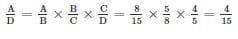RRB NTPC Mock Test - 16 (English) - Question 31

In the following figure Rectangle represents LED, Circle represents Inverter, Triangle represents Solar, and Square represents Battery. Which letter represents Solar and Battery but not Inverter?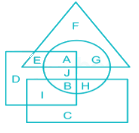Detailed Solution for RRB NTPC Mock Test - 16 (English) - Question 31

1) Rectangle represents LED.

2) Circle represents Inverter.

3) Triangle represents Solar.

4) Square represent Battery.

The letter that represents Solar and Battery but not Inverter i.e. letter that represents triangle and square but not circle which is the letter “E”.

Hence, “E” is the correct answer.

RRB NTPC Mock Test - 16 (English) - Question 32

From amongst the figures marked (A), (B), (C) and (D), select the figure which satisfies the same conditions of placement of the dots as in figure (X).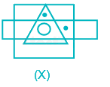Detailed Solution for RRB NTPC Mock Test - 16 (English) - Question 32

In figure (X), one of the dots lies in the region common to the square and the triangle only and the other dot lies in the region common to the square and the rectangle only. Only figure (D) consists of both types of regions.

Hence, figure (D) is the correct one.

RRB NTPC Mock Test - 16 (English) - Question 33

A 1000-metre-long train crosses a 500-metre-long bridge. If the speed of train is 30 km/hr, then what is the time taken (in seconds) to cross the bridge?

Detailed Solution for RRB NTPC Mock Test - 16 (English) - Question 33

We know that,

Distance = Speed × time

Total distance to travel = 1000 + 500 = 1500 m

Speed = 30 km/hr =30 × 5/18 = 25/3 m/s

Time taken = Distance/Speed = 1500/(25/3) = 180 second

RRB NTPC Mock Test - 16 (English) - Question 34

Which of the following bank partners with M1Xchange for MSME bill discounting?

Detailed Solution for RRB NTPC Mock Test - 16 (English) - Question 34
• Bank of Maharashtra partners with M1Xchange for MSME bill discounting
• Trade Receivables Discounting System is a digital platform to support micro, small and medium enterprises to get their bills financed at a competitive rate through an auction where multiple registered financiers can participate.
• Mynd Solutions is a technology management firm which runs the TReDS platform of M1Xchange.
• The main purpose of TReDS platform is to facilitate the smooth flow of liquidity in the system and make finance available at a competitive rate.
RRB NTPC Mock Test - 16 (English) - Question 35

If ‘-‘ means ‘+’, ‘+‘ means ‘×‘, ‘×‘ means ‘-‘ and ‘÷’ means ‘÷‘ then what is the value of:
18 + 16 ÷ 1 × 3 + 5 - 16 - 46 ÷ 23

Detailed Solution for RRB NTPC Mock Test - 16 (English) - Question 35

Changing operators as given format.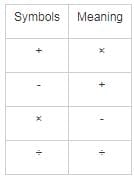Given expression: 18 + 16 ÷ 1 × 3 + 5 - 16 - 46 ÷ 23

After interchanging symbols: 18 × 16 ÷ 1 - 3 × 5 + 16 + 46 ÷ 23

= 18 × 16 - 3 × 5 + 16 + 2

= 288 - 15 + 16 + 2

= 288 + 18 - 15

= 291

RRB NTPC Mock Test - 16 (English) - Question 36

Find the wrong term in the following series.
HA, 9, FD, 8, DI, 6, CF, 6, BE, 5

Detailed Solution for RRB NTPC Mock Test - 16 (English) - Question 36

Given series: HA, 9, FD, 8, DI, 6, CF, 6, BE, 5

The given sequence is a combination (pair of the alphabet, square root of their respective position number).

The pair of the alphabet is followed by the square root of their respective position number.

HA → It’s respective position number is 81 and the square root of 81 is 9.

Similarly, FD → 64 and 8 is the square root of 64.

DI → 49 and the square root of 49 is 7 but DI is followed by 6.

Hence, the wrong term is 6 which is placed after DI.

RRB NTPC Mock Test - 16 (English) - Question 37

In which of the following cities The Central Office of the Reserve Bank was initially established?

Detailed Solution for RRB NTPC Mock Test - 16 (English) - Question 37
• The Central Office of the Reserve Bank was initially established in Calcutta.
• It was permanently moved to Mumbai in 1937.
• The Reserve Bank of India was established on April 1, 1935, in accordance with the provisions of the Reserve Bank of India Act, 1934.
• Shaktikanta Das is the current governor of RBI.
• Sir Osborne A. Smith was the first governor of RBI.
• CD Deshmukh was the first Indian governor of RBI .
RRB NTPC Mock Test - 16 (English) - Question 38

Who among the following is the first Indian bowler to take a hat-trick in test matches?

Detailed Solution for RRB NTPC Mock Test - 16 (English) - Question 38
• Harbhajan Singh is the first Indian bowler to take a hat-trick in test matches on 11 March 2001 in Kolkata.
• He got wickets of Ricky Ponting, Adam Gilchrist and Shane Warne.
• It was the 29th hattrick in test Cricket.
• Irfan Pathan is the second Indian bowler to take a hat-trick in test matches.
RRB NTPC Mock Test - 16 (English) - Question 39

Directions: In each question below is given a statement followed by two assumptions numbered I and II. You have to consider the statement and the following assumptions and decide which of the assumptions is implicit in the statement.

Statement: Web series have become a new source of entertainment for people nowadays.

Assumptions:
I.   Web series are new media available on the internet.
II.  People enjoy the content because it is not censored.

Detailed Solution for RRB NTPC Mock Test - 16 (English) - Question 39

In our country, web series are getting popular day after day and people are finding it more interesting. Assumption I is not implicit because it is just a definition of 'web series'. Assumption II is not implicit because there is no information provided in the statement regarding people liking web series because of the uncensored content.

RRB NTPC Mock Test - 16 (English) - Question 40

In the following question, by using which mathematical operators will the expression becomes correct?
17_13_6_2 = 16

Detailed Solution for RRB NTPC Mock Test - 16 (English) - Question 40

1) ×, +, - ⇒ 17 × 13 + 6 - 2 = 225

2) ×, -, + ⇒ 17 × 13 - 6 + 2 = 217

3) +, -, × ⇒ 17 + 13 - 6 × 2 = 18

4) -, +, × ⇒ 17 - 13 + 6 × 2 = 16

Hence, correct set of symbols is ‘-, +, ×’.

RRB NTPC Mock Test - 16 (English) - Question 41

If (a3 + b3) = 8125 and (a + b) = 25, find the value of (a2 + b2 – ab).

Detailed Solution for RRB NTPC Mock Test - 16 (English) - Question 41

∵ (a3 + b3) = (a + b)(a2 + b2 – ab)

⇒ 8125 = 25 × (a2 + b2 – ab)

⇒ (a2 + b2 – ab) = 8125/25

∴ (a2 + b2 – ab) = 325

RRB NTPC Mock Test - 16 (English) - Question 42

Arrange the given words in the sequence in which they occur in the dictionary.

i. Gentle
ii. Garage
iii. Game
iv. Garden

Detailed Solution for RRB NTPC Mock Test - 16 (English) - Question 42

On arranging the given word as per the dictionary order,

iii. Game

ii. Garage

iv. Garden

i. Gentle

Hence, “iii, ii, iv, i” is the correct order as per the dictionary.

RRB NTPC Mock Test - 16 (English) - Question 43

Select the option that depicts the following transparent sheet (Problem Figure) when folded at the dotted line shown.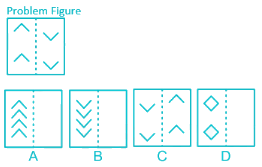Detailed Solution for RRB NTPC Mock Test - 16 (English) - Question 43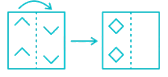Thus, the image that is formed after folding the paper is image D.

RRB NTPC Mock Test - 16 (English) - Question 44

Stem cutting propagation is used for the re-plantation of ________.

Detailed Solution for RRB NTPC Mock Test - 16 (English) - Question 44
• Stem cutting is an artificial method of plant propagation.
• In this method, the stem of a heavy plant is cut into small pieces.
• Each cutting must have at least 2-3 short internodes with axillary buds.
• The upper end of the cutting is given a transverse cut to mark the morphological apex of the stem.
• The obliquely cut basal and then planted in the soil under a suitable condition for the formation of adventitious roots.
• This method of propagation is used for Sugarcane, Rose, Grapes, Tea, etc.
RRB NTPC Mock Test - 16 (English) - Question 45

In which of the following Indian states did The Moplah Rebellion of 1921 took place?

Detailed Solution for RRB NTPC Mock Test - 16 (English) - Question 45
• The Moplah Rebellion of 1921 started in the Malabar district of Kerala.
• The Moplah tenants were Muslims and they agitated against the Hindu landlords and the British government.
• In the aftermath of this movement, the Suddhi Movement was created by the Arya Samaj.
• Sir C Shankaran Nair, a former President of the INC, criticised Gandhi’s support of the Khilafat Movement as one of the causes of the violence.
RRB NTPC Mock Test - 16 (English) - Question 46

Recently, SpiceJet has signed a pact with Emirates. Who among the following is the CEO of SpiceJet?

Detailed Solution for RRB NTPC Mock Test - 16 (English) - Question 46
• Ajay Singh is the CEO of SpiceJet.
• Recently, Spicejet announced the signing of an initial pact for codeshare partnership with Gulf carrier Emirates.
• Code-sharing allows an airline to book its passengers on its partner carriers and provide seamless travel to destinations where it has no presence.
• SpiceJet passengers from 51 domestic destinations will be able to access Emirates' network across the US, Europe, Africa and the Middle East.
• Headquarters of Spice Jet are in Gurugram.
RRB NTPC Mock Test - 16 (English) - Question 47

According to Pradhan Mantri Shram Yogi Mandhan (PM-SYM) Yojana, Government will provide a monthly pension of Rs. ________ to the employees of the unorganised sector.

Detailed Solution for RRB NTPC Mock Test - 16 (English) - Question 47
• Prime Minister Narendra Modi launched the Pradhan Mantri Shram Yogi Mandhan (PM-SYM) Yojana in Gandhinagar, Gujarat.
• The national pension scheme is for workers and laborers of the unorganized sector.
• It was announced in the interim budget in February this year.
• It will provide a monthly pension of 3000 rupees to the employees of the unorganised sector after 60 years of age.
RRB NTPC Mock Test - 16 (English) - Question 48

Annu Rani who won a silver medal at Asian Athletics Championship is related to which of the following sports?

Detailed Solution for RRB NTPC Mock Test - 16 (English) - Question 48
• Annu Rani won the silver medal in women’s Javelin throw.
• India won five medals including two silvers on Day 1 at Asian Athletics Championship
• India opened their account at the 23rd Asian Athletics Championship in Doha, Qatar on when Parul Chaudhary bagged a bronze medal in the women’s 5000m race.
• Avinash Sable won a silver medal in men’s 3000m steeplechase.
• MR Poovamma finished third in women’s 400m with bronze and Gavit Murali Kumar bagged another bronze medal for India in the 10,000m event.
RRB NTPC Mock Test - 16 (English) - Question 49

Which of the following numbers is irrational?

Detailed Solution for RRB NTPC Mock Test - 16 (English) - Question 49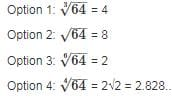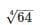cannot be expressed in terms of p/q, sois an irrational number.

RRB NTPC Mock Test - 16 (English) - Question 50

Pointing the man in the hospital, Ninad said, “he is the son of my grandfather’s only son.” How is Ninad related to that man? Ninad father has no daughter.

Detailed Solution for RRB NTPC Mock Test - 16 (English) - Question 50

From the given question,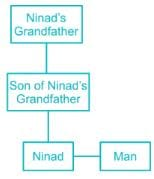Hence, Ninad is the brother of that man.

RRB NTPC Mock Test - 16 (English) - Question 51

Which of the following organization does not have its headquarters in Geneva, Switzerland?

Detailed Solution for RRB NTPC Mock Test - 16 (English) - Question 51
• The headquarters of the United Nations Education Scientific & Cultural Organisation (UNESCO) is located in Paris, France.
• It seeks to build peace through international cooperation in Education, Sciences and Culture.
• It was established on 4 November 1946.
• The current Director-General of UNESCO is Audrey Azoulay.
RRB NTPC Mock Test - 16 (English) - Question 52

If (1/2.315) = 0.4319, find the value of (1/0.0002315).

Detailed Solution for RRB NTPC Mock Test - 16 (English) - Question 52

Given,

⇒ (1/2.315) = 0.4319

Now,

∴ (1/0.0002315) = (1/2.315) × 10000 = 0.4319 × 10000 = 4319

RRB NTPC Mock Test - 16 (English) - Question 53

Directions: In the question below is a statement followed by two conclusions numbered I and II. You have to assume everything in the statement to be true, then consider the two conclusions together and decide which of them logically follows beyond a reasonable doubt from the information given in the statement.

Statement: Playing football daily for 1 hour can increase a person's life span by one year.

Conclusions:
I.   Moderate level of physical exercise is necessary for leading a healthy life.
II.   All people who do desk-bound jobs definitely suffer medical issues.

Detailed Solution for RRB NTPC Mock Test - 16 (English) - Question 53

In the statement, the benefit of playing football is mentioned, but no information is given regarding the health of a person doing a desk-bound job. Hence only conclusion I follows.

RRB NTPC Mock Test - 16 (English) - Question 54

If cream‘’ means ‘ chocolate’, ‘bread’ means ‘butter’, ‘milk’ means ‘tea’, ‘ice’ means ‘cold’ and ‘Lemon’ means Soda than what is ice cream?

Detailed Solution for RRB NTPC Mock Test - 16 (English) - Question 54

Here cream = chocolate, bread = butter, milk = tea, ice = cold and lemon = soda therefore ice cream = cold chocolate

Hence, cold chocolate is correct answer.

RRB NTPC Mock Test - 16 (English) - Question 55

Match the following with their correct places:

1. Arid Zone Research Institute i) Kolkata
2. Indian Anthropology Survey ii) Jodhpur
3. Wildlife Institute of India iii)Dehradun
4. Tropical Forest Research Institute iv) Jabalpur

Detailed Solution for RRB NTPC Mock Test - 16 (English) - Question 55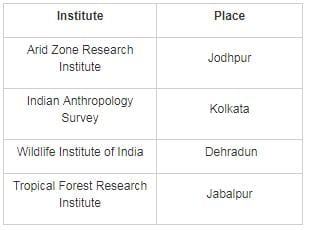RRB NTPC Mock Test - 16 (English) - Question 56

A, B and C can do a job working alone in 50, 75 and 20 days respectively. They all work together for 4 days, then C quits. How many days will A and B take to finish the rest of the job?

Detailed Solution for RRB NTPC Mock Test - 16 (English) - Question 56

Suppose unit work = 300 units (LCM of 50, 75 & 20)

∴ Work done by A in one day = 300/50 = 6

Work done by B in one day = 300/75 = 4

Work done by C in one day = 300/20 = 15

∴ Work done by (A + B + C) in 4 days = 4 × (6 + 4 + 15) = 100

C quits after 4 days.

∴ Remaining work after 4 days = 300 – 100 = 200 units

∴ Time taken by A and B together to complete 200 units = 200/(6 + 4) = 20 days

RRB NTPC Mock Test - 16 (English) - Question 57

Calangute is famous for its beaches. In which of the following states this beach is situated?

Detailed Solution for RRB NTPC Mock Test - 16 (English) - Question 57
• Calangute is a town in Goa.
• Other famous places of Goa are Kola, Vagator, Anjuna Beach, St. Cathedral Church, merdal, Terekhol.
• Pramod Sawant is Chief Minister of Goa
• Goa’s Capital: Panaji
• Rajya Sabha seats in Goa: 1
• Lok Sabha seats in Goa: 2
RRB NTPC Mock Test - 16 (English) - Question 58

The Panchsheel Treaty was signed in which of the following year?

Detailed Solution for RRB NTPC Mock Test - 16 (English) - Question 58
• The Panchsheel or ‘Five Principles of Peaceful Existence’ was signed between India and China in 1954.

This agreement stated the five principles as:

• Mutual respect for each other's territorial integrity and sovereignty.
• Mutual non-aggression.
• Mutual non-interference in each other's internal affairs.
• Equality and cooperation for mutual benefit.
• Peaceful co-existence.

• These five principles form the basis of the relationship between India and China.
RRB NTPC Mock Test - 16 (English) - Question 59

City U is 9 km towards the east of City V.
City W is 5 km towards the south of City U.
City X is 3 km towards the west of City W.
City Y is 5 km towards the north of City X.
City Z is 7 km towards the south of City X.

Q. Which of the cities lie on a straight line?

Detailed Solution for RRB NTPC Mock Test - 16 (English) - Question 59

The given information can be represented as follows,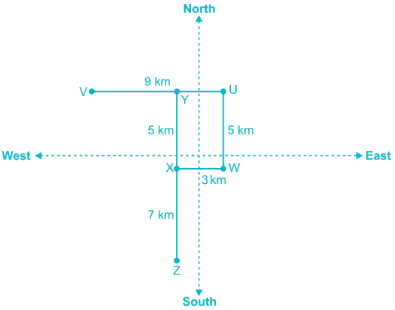So, cities XYZ are in line.

RRB NTPC Mock Test - 16 (English) - Question 60

According to a study by NASA, what was the first molecule formed in space?

Detailed Solution for RRB NTPC Mock Test - 16 (English) - Question 60
• NASA detects Universe’s first molecule in space i.e., the Helium hydride ion
• Scientists have detected the most ancient type of molecule in our universe in space for the first time ever.
• It was formed when falling temperatures in the young universe allowed recombination of the light elements produced in the Big Bang.
• At that time, ionised hydrogen and neutral helium atoms reacted to form HeH+.
RRB NTPC Mock Test - 16 (English) - Question 61

Find the value of ‘?’ in the following question?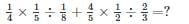Detailed Solution for RRB NTPC Mock Test - 16 (English) - Question 61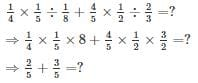⇒ ? = 1

RRB NTPC Mock Test - 16 (English) - Question 62

Which of the following country hosted the Special Olympics World Games 2019?

Detailed Solution for RRB NTPC Mock Test - 16 (English) - Question 62
• Special Olympics World Games 2019 held at UAE from March 14 to March 21.
• It is a multi-sport event for athletes with intellectual disabilities in the tradition of the Special Olympics movement.
• India won 368 medals (85 gold, 154 Silver, and 129 Bronze) at the game.
• The next Special Olympic Games will take place in Sweden in 2021.
RRB NTPC Mock Test - 16 (English) - Question 63

Select the odd letters from the given alternatives.

Detailed Solution for RRB NTPC Mock Test - 16 (English) - Question 63

Here according to the position of each letter we can say that,

U + 3 = X – 4 = T

L + 3 = O – 5 = J

K + 3 = N – 4 = J

D + 3 = G – 4 = C

Therefore, we can say that LOJ is the answer.

RRB NTPC Mock Test - 16 (English) - Question 64

If (3a/m + b/n) =4 and (a/m - b/n) = 4, Find the values of ‘a’ and ‘b’ respectively.

Detailed Solution for RRB NTPC Mock Test - 16 (English) - Question 64

3a/m + b/n = 4       ----(1)

a/m - b/n = 4      ----(2)

4a/m = 8

∴ a = 2m

Putting the value of a in eq (2)

2m/m - b/n = 4

⇒ b/n = 2 - 4 = -2

∴ b = -2n

RRB NTPC Mock Test - 16 (English) - Question 65

In the following question, select the number which can be placed at the sign of question mark (?) from the given alternatives.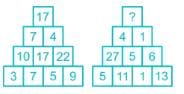Detailed Solution for RRB NTPC Mock Test - 16 (English) - Question 65

In first figure,

⇒ [(3 × 7) – 1] ÷ 2 = 10; [(7 × 5) – 1] ÷ 2 = 17; [(5 × 9) – 1] ÷ 2 = 22;

⇒ Difference of sum of digits of 17 & 10 → 8 – 1 = 7

⇒ Difference of sum of digits of 17 & 22 → 8 – 4 = 4

Product of 7 & 4 – sum of 7 & 4 → 28 – 11 = 17

Similarly,

In 2nd figure,

⇒ [(5 × 11) – 1] ÷ 2 = 27; [(11 × 1) – 1] ÷ 2 = 5; [(1 × 13) – 1] ÷ 2 = 6;

⇒ Difference of sum of digits of 27 & 5 → 9 – 5 = 4

⇒ Difference of sum of digits of 6 & 5 → 6 – 5 = 1

Product of 1 & 4 – sum of 1 & 4 → 4 – 5 = – 1

Hence, “-1” is the missing number.

RRB NTPC Mock Test - 16 (English) - Question 66

Select an appropriate figure from the four options that would complete the figure.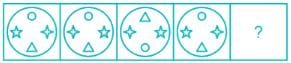Detailed Solution for RRB NTPC Mock Test - 16 (English) - Question 66

The symbols interchange positions horizontally in the next figure,

The symbols interchange positions vertically in the next figure,

The symbols interchange positions both horizontally and vertically in the next figure and this three process gets continued, thus we get option 3 as the answer.

RRB NTPC Mock Test - 16 (English) - Question 67

A boy completes 90 rounds of a garden in 1 hour. On an average, how many rounds will he complete in 16 minute?

Detailed Solution for RRB NTPC Mock Test - 16 (English) - Question 67

The boy completes 90 rounds in 1 hr (60 min)

In 1 minute, he will complete = 90/60 = 1.5 rounds

∴ In 16 min, he will complete = 16 × 1.5 = 24 rounds

RRB NTPC Mock Test - 16 (English) - Question 68

If the difference between a number and 37.5% of the number is 45, find the number.

Detailed Solution for RRB NTPC Mock Test - 16 (English) - Question 68

Let the number be ‘x’

⇒ x – 37.5% of x = 45

⇒ x – (3/8)x = 45

⇒ (5/8)x = 45

⇒ x = 8/5 × 45 = 72

∴ The number is 72

RRB NTPC Mock Test - 16 (English) - Question 69

The divisor is 50 times the quotient and 10 times the remainder. If the quotient is 32, what is the dividend?

Detailed Solution for RRB NTPC Mock Test - 16 (English) - Question 69

Let the divisor be x

Quotient = x/50

Remainder = x/10

x/50 = 32 ⇒ x = 1600

The number/dividend is (Divisor × Quotient) + Remainder

Number = x2/50 + x/10

Dividend = (16002/50) + 1600/10 = 51360

RRB NTPC Mock Test - 16 (English) - Question 70

Which of the following disease is also known as Dumdum fever?

Detailed Solution for RRB NTPC Mock Test - 16 (English) - Question 70
• Kala Azar is also known as Dumdum fever.
• It is caused by protozoan parasites of the genus Leishmania.
• It affects the internal organs, particularly the liver, spleen, bone marrow and lymph nodes.
• The first oral drug found to be effective in treating kala-azar is miltefosine.
RRB NTPC Mock Test - 16 (English) - Question 71

The term ‘Ebola’ in Ebola virus has been derived from ________.

Detailed Solution for RRB NTPC Mock Test - 16 (English) - Question 71
• The term Ebola has been derived from river Ebola which is a tributary of the Congo River, in the northern Democratic Republic of the Congo.
• The river course is thought to be a place where the 1976 Zaire Ebola virus outbreak occurred.
• The Ebola virus causes an acute, serious illness which is often fatal if untreated.
• It is transmitted to people from wild animals and spreads in the human population through human-to-human transmission.
RRB NTPC Mock Test - 16 (English) - Question 72

In a row of 50 persons, position of Lexa is 34th from the left side. What is position of Lexa from the right side of the row?

Detailed Solution for RRB NTPC Mock Test - 16 (English) - Question 72

Position of Lexa from the right side = Total no. of persons – Position of Lexa from the left side + 1 → 50 – 34 + 1 → 51 – 34 → 17

Hence, Lexa is 17th from the right side of the row.

RRB NTPC Mock Test - 16 (English) - Question 73

Of three positive numbers, the ratio of 1st and 2nd is 7 : 3, that of 2nd and 3rd is 4 : 5. The product of 1st and 3rd is 10500. What is the sum of the three numbers?

Detailed Solution for RRB NTPC Mock Test - 16 (English) - Question 73

Let,

Let the three numbers be x, y and z

x/y = 7/3

y/z = 4/5

x = 7y/3      ----- (1)

z = 5y/4       ----- (2)

Given, xz = 10500     ----- (3)

Put value of (1) and (2) in (3)

35y2/12 = 10500

y2 = 3600

y = 60

x = 140

z = 75

Sum of three numbers = 140 + 60 + 75 = 275

RRB NTPC Mock Test - 16 (English) - Question 74

Which of the following device is used to measure altitude and velocity of clouds?

Detailed Solution for RRB NTPC Mock Test - 16 (English) - Question 74
• Nephoscope is a device used to measure altitude, direction, and velocity of clouds.
• It uses the principle of reflection of light by the cloud to measure them.
• The distance of cloud = (speed of light × duration of delay due to reflection) / 2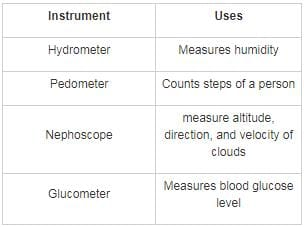RRB NTPC Mock Test - 16 (English) - Question 75

The mean of a distribution is 21 and the standard deviation is 7. What is the value of the coefficient variation?

Detailed Solution for RRB NTPC Mock Test - 16 (English) - Question 75

We know that coefficient of variation = standard deviation/mean = 7/21 = 0.33 = 0.3333 × 100 = 33.33%

RRB NTPC Mock Test - 16 (English) - Question 76

Who among the following has the right to set up a High Court in any Union Territory?

Detailed Solution for RRB NTPC Mock Test - 16 (English) - Question 76
• Parliament may by law constitute a High Court for a Union territory or declare any court in any such territory to be a High Court for all or any of the purposes of this Constitution.
• Only Delhi is the only Union Territory that has its own high court.
• Calcutta High Court is the oldest High Court in India established on 1 July 1862.
RRB NTPC Mock Test - 16 (English) - Question 77

At a certain rate per annum, the simple interest on a sum of money for one year is Rs. 260 and the compound interest on the same sum for two years is Rs. 540.80. The rate of interest per annum is

Detailed Solution for RRB NTPC Mock Test - 16 (English) - Question 77

Formula :
SI = (P × r × t)/100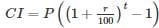Where p is the sum, r is rate and t is the time in years.
Given, simple interest on a sum of money for one year is Rs. 260 and the compound interest on the same sum for two years is Rs. 540.80.
⇒ 260 = (p × r)/100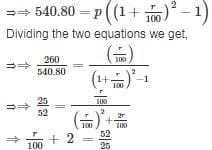⇒ r = 200/25
⇒ r = 8

RRB NTPC Mock Test - 16 (English) - Question 78

In the following figure Rectangle represents triangle, Circle represents square, Triangle represents rectangle, and Square represents circle. Which letter represent circle and rectangle?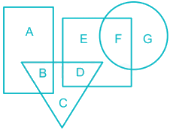Detailed Solution for RRB NTPC Mock Test - 16 (English) - Question 78

1) Rectangle represent triangle.

2) Circle represent square.

3) Triangle represent rectangle.

4) Square represent circle.

The letter that represents circle and rectangle i.e. letter that represents the intersection of Square and Triangle which is letter “D”.

Hence, D” is the correct answer.

RRB NTPC Mock Test - 16 (English) - Question 79

Which was India’s first Earth observation satellite?

Detailed Solution for RRB NTPC Mock Test - 16 (English) - Question 79
• Bhaskara-I was India’s first Earth observation satellite launched on June 07, 1979.
• It was the First Experimental Remote Sensing Satellite built in India.
• The onboard TV camera imageries were used in the field of Hydrology and Forestry.
• The satellite was named after ancient Indian mathematicians Bhāskara I.
RRB NTPC Mock Test - 16 (English) - Question 80

Choose the odd number among the numbers given below.

Detailed Solution for RRB NTPC Mock Test - 16 (English) - Question 80

667, 319 and 145 are multiples of 29 whereas 234 is not.

Hence, 234 is the odd one out.

RRB NTPC Mock Test - 16 (English) - Question 81

The ratio of expenditure and savings is 4 : 5. If the income increases by 20% and the savings increases by 6%, then by how much percent should his expenditure increase.

Detailed Solution for RRB NTPC Mock Test - 16 (English) - Question 81

⇒ Let his expenditure and savings be 40 and 50 respectively.

⇒ Then his income is 40 + 50 = 90

⇒ Income increase by 20% then,

⇒ New income = 90 × (120/100) = 108

⇒ Saving increases by 6% then,

⇒ New savings = 50 × (106/100) = 53

⇒ New expenditure = 108 – 53 = 55

⇒ Percentage increase in expenditure = (55 − 40) × (100/40) = 37.5%

RRB NTPC Mock Test - 16 (English) - Question 82

How much water must be added to 50 ml of alcohol to make a solution that contains 50% alcohol?

Detailed Solution for RRB NTPC Mock Test - 16 (English) - Question 82

Let the water added be x ml

Total solution = (x + 50) ml

% of alcohol = 50%

⇒ 50/(x + 50) × 100= 50

⇒ x = 50 ml

RRB NTPC Mock Test - 16 (English) - Question 83

The base of a conical tent is 19.2 m in diameter and height is 2.8 m. The area of canvas required to put such tent is:

Detailed Solution for RRB NTPC Mock Test - 16 (English) - Question 83

We have 2 × r = 19.2

⇒ r = 19.2/2

⇒ r = 9.6 m

Also we have slant height l as

⇒ l2 = r2 + h2

⇒ l2 = 9.6 × 9.6 + 2.8 × 2.8

⇒ l2 = 92.16 + 7.84

⇒ l2 = 100

⇒ l = 10

Hence, area of tent required = πrl

= (22/7) × 9.6 × 10 = 301.71

RRB NTPC Mock Test - 16 (English) - Question 84

The total percentage of illiterates in all the four cities is _______ (round to one decimal place).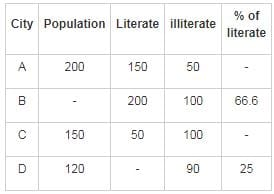Detailed Solution for RRB NTPC Mock Test - 16 (English) - Question 84

Total population in city B = 200 + 100 = 300

Total population in all the four cities = 200 + 300 + 150 + 120 = 770

Total population of illiterates in all the four cities = 50 + 100 + 100 + 90 = 340

∴ total percentage of illiterates in all the four cities = 340/770 × 100 = 44.155% ≈ 44.2%

RRB NTPC Mock Test - 16 (English) - Question 85

Bum La Pass is located in ___________

Detailed Solution for RRB NTPC Mock Test - 16 (English) - Question 85
• Bum La/Bomdila Pass is located in Tawang district of Arunachal Pradesh.
• This pass connects India and China.
• Through this pass, the Chinese army invaded India during the 1962 Sino-Indian War.
• It is one of the five officially agreed Border Personnel Meeting points between the Indian Army and the People's Liberation Army of China.
• अरुणाचल प्रदेश के दर्रे
Trick — “अरुण ने* बीज* बो दिया”

1. बो——-बोमडिला दर्रा/बम ला
2. दि——-दिफू दर्रा
3. या——-यांग्याप दर्रा

RRB NTPC Mock Test - 16 (English) - Question 86

Who among the following discovered Penicillin?

Detailed Solution for RRB NTPC Mock Test - 16 (English) - Question 86
• Penicillin was discovered by Alexander Fleming in 1928.
• It is the first and most widely used antibiotic agent.
• It is derived from Penicillium fungi which are considered as the most important natural source of Penicillin.
• It is used in the treatment of health problems like throat infections, meningitis, syphilis.
RRB NTPC Mock Test - 16 (English) - Question 87

A shopkeeper announced a discount of 30%, such that he earned a profit of 16.67% on all his products. If the profit earned is Rs. 250, what is its marked price?

Detailed Solution for RRB NTPC Mock Test - 16 (English) - Question 87

Let the marked price of the product be Rs. ‘x’

⇒ Discount = 30% of x = 0.3x

⇒ Selling price = Marked price – Discount = x – 0.3x = 0.7x

Now,

⇒ Profit earned = 16.67% of Cost Price = CP/6

Also,

⇒ Selling price = Cost price + Profit

⇒ 0.7x = CP + (CP/6)

⇒ 0.7x = (7/6)CP

⇒ CP = 0.6x

⇒ Profit earned = CP/6 = 0.6x/6 = 0.1x

As, Profit earned = Rs. 250

⇒ 0.1x = 250

⇒ x = 250/0.1 = Rs. 2500

∴ Marked price of product is Rs. 2500

RRB NTPC Mock Test - 16 (English) - Question 88

Which of the following countries is not a part of G7 group?

Detailed Solution for RRB NTPC Mock Test - 16 (English) - Question 88
• The Group of Seven (G7) is a group consisting of Canada, France, Germany, Italy, Japan, the United Kingdom, and the United States.
• These are the most advanced economies of the world and represent about 58% of the net global wealth.
• The 44th G7 summit took place between 8-9 June 2018 in Canada.
• In March 2019, Italy has joined the china’s mega connectivity scheme, One Belt One Road (OBOR) and has become the first G7 country to do so.
• G-7 Countries names-:
GK Trick-: drink JUICE with GF (girlfriend)
1) Japan
2) USA
3) Italy
5) England
6) Germany
7) France
RRB NTPC Mock Test - 16 (English) - Question 89

Galvanized iron sheets are coated with __________.

Detailed Solution for RRB NTPC Mock Test - 16 (English) - Question 89
• Galvanized iron sheets are coated with Zinc.
• Galvanization is the process of applying a protective zinc coating to steel and iron in order to prevent it from rusting.
• Zinc weathers at a very slow rate, so the coating generally has a long life.
• Zinc has a greater electronegativity than iron and hence provides cathodic protection to the steel.
RRB NTPC Mock Test - 16 (English) - Question 90

BOD is the amount of dissolved oxygen used by microorganisms. BOD stands for _________.

Detailed Solution for RRB NTPC Mock Test - 16 (English) - Question 90
• BOD stands for Biochemical Oxygen Demand.
• It is the amount of dissolved oxygen required by biological organisms to break down organic material present in the water over a specific time period.
• More organic matter in water results in greater BOD; the greater the BOD will result in the lower the amount of dissolved oxygen available for higher animals such as fishes.
• BOD is also used as a gauge to measure the effectiveness of wastewater treatment plants.
RRB NTPC Mock Test - 16 (English) - Question 91

When did Indian Space Research Organisation (ISRO) form?

Detailed Solution for RRB NTPC Mock Test - 16 (English) - Question 91
• K. Sivan is the current chairman of the Indian Space Research Organisation (ISRO).
• It is the space agency of the Government of India.
• Formation: 15 August 1969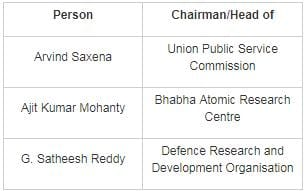RRB NTPC Mock Test - 16 (English) - Question 92

Which country’s Presidential election did Comedian Zelensky win?

Detailed Solution for RRB NTPC Mock Test - 16 (English) - Question 92
• Comedian Zelensky wins the presidential election of Ukraine.
• Exit polls indicated that he is going to take 73 per cent of the votes while incumbent Petro Poroshenko securing 25 per cent.
• Zelensky's only previous political role has been playing the President on television.
• Capital of Ukraine is Kyiv and Currency is Ukrainian hryvnia.
RRB NTPC Mock Test - 16 (English) - Question 93

A series is given with one term missing. Select the correct alternative from the given ones that will complete the series.
315, 316, 158, 79, 80, 40, 20, 10, 5, 6, ?

Detailed Solution for RRB NTPC Mock Test - 16 (English) - Question 93

The relation among given numbers is that when a number is even, we divide by 2 to get the next number. When the number is odd, we add one to get the next number:

315 → Odd → 315 + 1 = 316

316 → Even → 316 ÷ 2 = 158

158 → Even → 158 ÷ 2 = 79

79 → Odd → 79 + 1 = 80

80 → Even → 80 ÷ 2 = 40

40 → Even → 40 ÷ 2 = 20

20 → Even → 20 ÷ 2 = 10

10 → Even → 10 ÷ 2 = 5

5 → Odd → 5 + 1 = 6

6 → Even → 6 ÷ 2 = 3

Hence, “3” will complete the series.

RRB NTPC Mock Test - 16 (English) - Question 94

Some image in computers are stored in PNG format. PNG stands for ________.

Detailed Solution for RRB NTPC Mock Test - 16 (English) - Question 94
• PNG stands for Portable Network Graphics.
• They were created to replace Graphics Interchange Format (GIF) because the patent for GIF was owned by one company.
• Like GIF images, PNG also has the ability to display transparent backgrounds.
• These type of images are capable of containing 24-bit RGB colour palettes and Grayscale images.
RRB NTPC Mock Test - 16 (English) - Question 95

The height of an equilateral triangle is 15 cm. The area of the triangle is

Detailed Solution for RRB NTPC Mock Test - 16 (English) - Question 95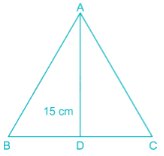We know that each angle in an equilateral triangle is 60°.

So, applying trigonometric formulae in this question we get,

In ΔABD,

⇒ √3 = 15/BD

⇒ BD = 5 √3 cm.

Since, AD is the height of an equilateral triangle; it divides BC into two equal parts, so,

BC = 2 × BD

⇒ BC = 10 √3

Now Area of a Triangle = ½ × Base × Height

⇒ Area = ½ × BC × AD

⇒ Area = ½ × 10 √3 × 15

⇒ Area = 75 √3 cm2

RRB NTPC Mock Test - 16 (English) - Question 96

The difference between the simple interest on Rs. X at 10% p.a. and the simple interest on Rs. Y at 8% p.a. after 3 years is Rs. 2100. What will be the difference after 7 years?

Detailed Solution for RRB NTPC Mock Test - 16 (English) - Question 96

As,

⇒ Simple interest = (Principal × Rate × Time)/100

Now,

⇒ Simple interest on Rs. X at 10% p.a. after 3 years = (X × 10 × 3)/100 = 0.3X

⇒ Simple interest on Rs. Y at 8% p.a. after 3 years = (Y × 8 × 3)/100 = 0.24Y

⇒ Given difference 2100

⇒ 0.3X – 0.24Y = 2100

⇒ 0.1X – 0.08Y = 700 ----(1)

Now,

⇒ Simple interest on Rs. X at 10% p.a. after 7 years = (X × 10 × 7)/100 = 0.7X

⇒ Simple interest on Rs. Y at 8% p.a. after 7 years = (Y × 8 × 7)/100 = 0.56Y

⇒ Required difference = 0.7X – 0.56Y = 7(0.1X – 0.08Y)

Substituting from (1),

∴ Required difference = 7 × 700 = Rs. 4900

RRB NTPC Mock Test - 16 (English) - Question 97

Who among the following has been appointed as India's next High Commissioner to South Africa?

Detailed Solution for RRB NTPC Mock Test - 16 (English) - Question 97
• Jaideep Sarkar has been appointed as India's next High Commissioner to South Africa.
• Sarkar, a 1987-batch Indian Foreign Service officer, is currently serving as India's ambassador in Bhutan.
• He served in Indian Missions in Tokyo, Seoul and Bangladesh as well.
• The government also appointed Suhel Ajaz Khan, presently serving as India's Deputy Chief of Mission in Saudi Arabia, as the next Ambassador to Lebanon.
RRB NTPC Mock Test - 16 (English) - Question 98

Direction: Read the following information carefully and answer the questions that follow:

A, B, C, D, E are five boys sitting in a line facing south. While M, N, O, P and Q are five girls sitting in a second line parallel to a first line facing north and they are facing each other. B who is just next to the left of D is to opposite Q. C and N are sitting at the extreme end diagonally opposite to each other. E is opposite to O who is just next right of M. P who is just to the left of Q, is opposite to D. M is at one end of the line.

Q. Who is opposite to  M?

Detailed Solution for RRB NTPC Mock Test - 16 (English) - Question 98

Given:

i) Boys: A, B, C, D, E are five boys sitting in a line facing south.

ii) Girls: M, N, O, P and Q are five girls sitting in second line parallel to first line facing north.

iii) They are facing each other.

1. B who is just next to the left of D, is opposite Q.

2. C and N are sitting at the extreme end diagonally opposite to each other.

3. P who is just to the left of Q is opposite to D.

4. M is at one end of the line.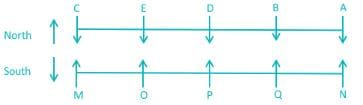Hence, M is sitting opposite to C.

RRB NTPC Mock Test - 16 (English) - Question 99

Direction: Read the following information carefully and answer the questions that follow:

A, B, C, D, E are five boys sitting in a line facing south. While M, N, O, P and Q are five girls sitting in a second line parallel to a first line facing north and they are facing each other. B who is just next to the left of D is to opposite Q. C and N are sitting at the extreme end diagonally opposite to each other. E is opposite to O who is just next right of M. P who is just to the left of Q, is opposite to D. M is at one end of the line.

Q. How many people are sitting to the right of the person facing N?

Detailed Solution for RRB NTPC Mock Test - 16 (English) - Question 99

Given:

i) Boys: A, B, C, D, E are five boys sitting in a line facing south.

ii) Girls: M, N, O, P and Q are five girls sitting in second line parallel to first line facing north.

iii) They are facing each other.

1. B who is just next to the left of D, is opposite Q.

2. C and N are sitting at the extreme end diagonally opposite to each other.

3. P who is just to the left of Q is opposite to D.

4. M is at one end of the line.Hence, A is facing N and persons are sitting to the right of A.

RRB NTPC Mock Test - 16 (English) - Question 100

If in a certain code language CAPSIZED is coded following a certain pattern. The coded word is GUX?SNSO. Choose the correct letter to replace the question mark.

Detailed Solution for RRB NTPC Mock Test - 16 (English) - Question 100

The pattern for this code is as follows,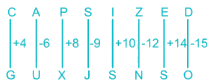Here, the values are in increasing order of non-prime numbers starting from 4 from the left.

Hence, J will be there in the place of question mark.

## RRB NTPC Mock Tests Series

20 tests
 Use Code STAYHOME200 and get INR 200 additional OFF Use Coupon Code
Information about RRB NTPC Mock Test - 16 (English) Page
In this test you can find the Exam questions for RRB NTPC Mock Test - 16 (English) solved & explained in the simplest way possible. Besides giving Questions and answers for RRB NTPC Mock Test - 16 (English), EduRev gives you an ample number of Online tests for practice

20 tests